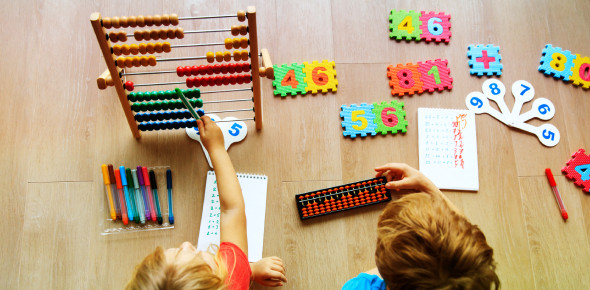# Maths Quiz: Can You Answer All The Questions In 2 Minutes?

Approved & Edited by ProProfs Editorial Team
At ProProfs Quizzes, our dedicated in-house team of experts takes pride in their work. With a sharp eye for detail, they meticulously review each quiz. This ensures that every quiz, taken by over 100 million users, meets our standards of accuracy, clarity, and engagement.
| Written by 700020
7
700020
Community Contributor
Quizzes Created: 2 | Total Attempts: 34,999
Questions: 10 | Attempts: 34,819SettingsWelcome to this awesome 'Maths Quiz' that we've designed below for you. Mathematics is an enjoyable thing to learn if you open your mind to it. Do you consider yourself a math whiz such that you can get answers to some calculations without using a calculator? If you said a big yes to me, then this easy quiz is for you. Try answering it in less than two minutes. Let's see how much you can score. All the best!

• 1.

### What Is 24 X 2?

• A.

45

• B.

Pass

• C.

26

• D.

48

• E.

125

D. 48
Explanation
The correct answer is 48 because when you multiply 24 by 2, you get 48.

Rate this question:

• 2.

### √25= ______

5
Explanation
The square root of 25 is equal to 5.

Rate this question:

• 3.

### What is 2 to the power 4?

16
Explanation
The question is asking for the value of 2 raised to the power of 4. This means multiplying 2 by itself 4 times. When we do this calculation, we get the result of 16.

Rate this question:

• 4.

### 1318 ÷ 2?

659
Explanation
When dividing 1318 by 2, we are essentially splitting 1318 into two equal parts. Each part will have a value of half of 1318, which is 659. Therefore, the answer is 659.

Rate this question:

• 5.

### 0.75 is the same as?

• A.

7.5%

• B.

75%

• C.

750%

• D.

0.075%

• E.

Pass

B. 75%
Explanation
The given question asks for the equivalent value of 0.75. To convert a decimal to a percentage, we move the decimal point two places to the right and add a percent sign. Therefore, 0.75 is equal to 75%.

Rate this question:

• 6.

### What is 12 squared?

144
Explanation
The question is asking for the result of squaring the number 12. To square a number means to multiply it by itself. So, 12 squared is equal to 12 multiplied by 12, which is 144.

Rate this question:

• 7.

### (x+5)(x-10)= ________.

• A.

X2 -25

• B.

X2 -50x-50

• C.

X2 -5x-50

• D.

X2 +5x-50

C. X2 -5x-50
Explanation
The given expression represents the product of two binomials. Using the FOIL method, we can expand the expression to obtain x^2 - 10x + 5x - 50, which simplifies to x^2 - 5x - 50. Therefore, the correct answer is x^2 - 5x - 50.

Rate this question:

• 8.

### (5-4)-(12+18-2)= _________.

• A.

27

• B.

-27

• C.

-28

• D.

30

B. -27
Explanation
The given expression involves subtraction and addition of numbers. First, we calculate the sum of the numbers inside the parentheses: (12+18-2) = 28. Then, we perform the subtraction outside the parentheses: (5-4) = 1. Finally, we subtract the sum from the subtraction: 1 - 28 = -27.

Rate this question:

• 9.

### √5 = ______.

• A.

2.2360

• B.

2.2659

• C.

4.2367

• D.

0.3241

A. 2.2360
Explanation
The square root of 5 is approximately equal to 2.2360.

Rate this question:

• 10.

### 122 X 4 = ______.

• A.

444

• B.

448

• C.

488

• D.

490Back to top